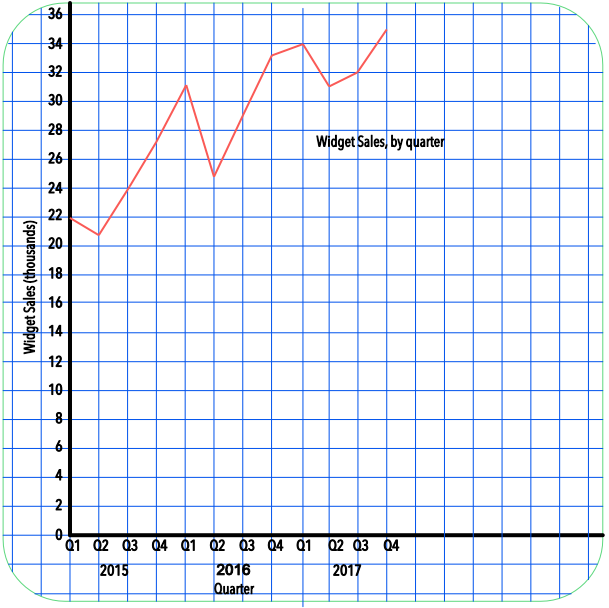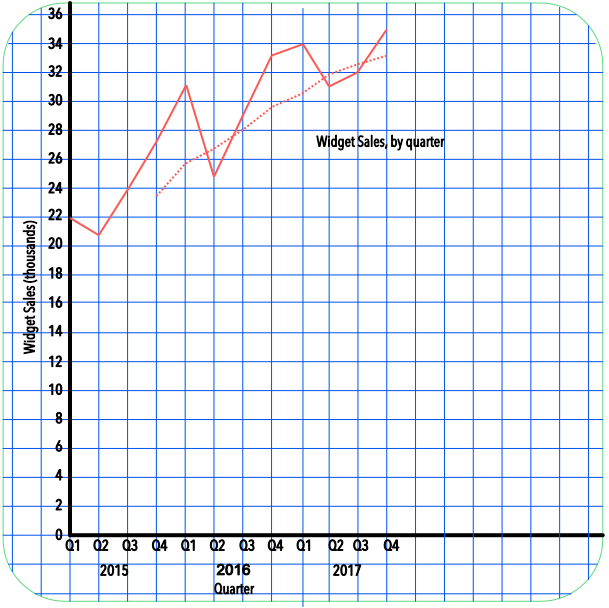Time Series Data

# Time Series Data

GCSE(F), GCSE(H),

A graph that shows Time Series Data is a plot of value against time, with time shown along the bottom of the graph.

More than one set of data may be plotted on a graph: for example, plotting a comparison of rainfall between two different places.

Time series data can be used to determine a Moving Average that smooths out the peaks and troughs of a graph so that any Trend, or movement, can be determined. A moving average uses the mean of the number of periods: for example, a three-month moving average will add the values for three consecutive months and divide by 3. A moving average can only be plotted when the number of periods in the moving averages are available.

## Examples

1. The sales of widgets are noted on a table for each Quarter over the previous three years. Plot the time series on a graph.

Quarter2015 Q12015 Q22015 Q32015 Q42016 Q12016 Q22016 Q32016 Q42017 Q12017 Q22017 Q32017 Q4
Widgets (thousands)222124273125293334313235

Answer:Remember to title the graph, and to name the axes and provide scales.

2. For the sales of widgets, above, plot the 4-quarter moving average on the same graph.

Answer:Moving average for 2015 Q4 = (22 + 21 + 24 + 27) ÷ 4 = 23.5

Moving average for 2016 Q1 = (21 + 24 + 27 + 31) ÷ 4 = 25.8

Moving average for 2016 Q2 = (24 + 27 + 31 + 25) ÷ 4 = 26.7 etc..

Quarter2015 Q12015 Q22015 Q32015 Q42016 Q12016 Q22016 Q32016 Q42017 Q12017 Q22017 Q32017 Q4
Widgets (thousands)222124273125293334313235
4-Quarter Mov. Ave.23.525.826.72829.530.331.832.533